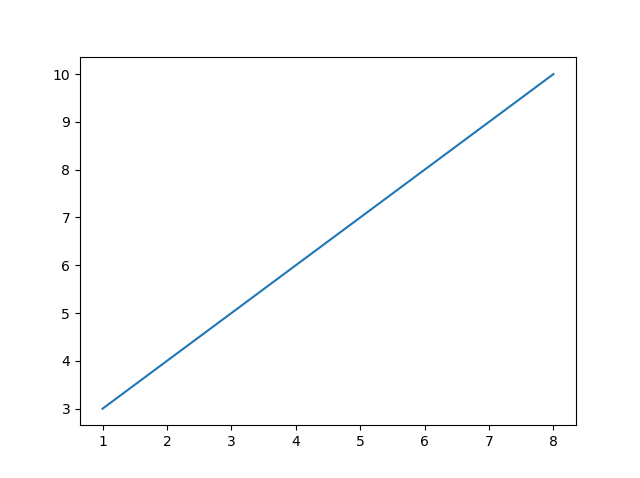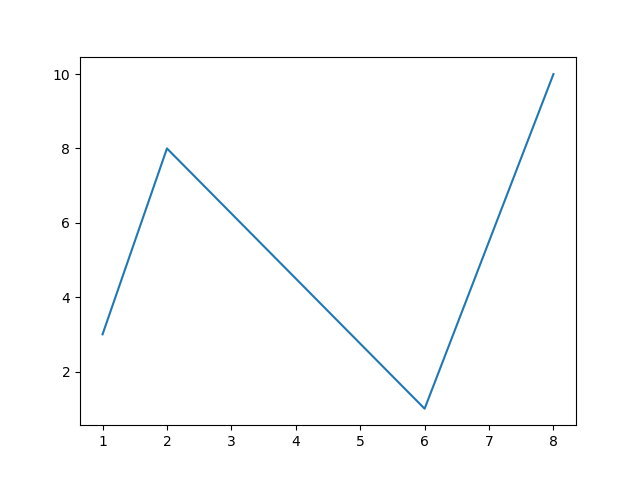## Python Tutorial

Python HOME Python Intro Python Get Started Python Syntax Python Comments Python Variables Python Data Types Python Numbers Python Casting Python Strings Python Booleans Python Operators Python Lists Python Tuples Python Sets Python Dictionaries Python If...Else Python While Loops Python For Loops Python Functions Python Lambda Python Arrays Python Classes/Objects Python Inheritance Python Iterators Python Polymorphism Python Scope Python Modules Python Dates Python Math Python JSON Python RegEx Python PIP Python Try...Except Python User Input Python String Formatting

## File Handling

Python File Handling Python Read Files Python Write/Create Files Python Delete Files

## Python Modules

NumPy Tutorial Pandas Tutorial SciPy Tutorial Django Tutorial

## Python Matplotlib

Matplotlib Intro Matplotlib Get Started Matplotlib Pyplot Matplotlib Plotting Matplotlib Markers Matplotlib Line Matplotlib Labels Matplotlib Grid Matplotlib Subplot Matplotlib Scatter Matplotlib Bars Matplotlib Histograms Matplotlib Pie Charts

## Machine Learning

Getting Started Mean Median Mode Standard Deviation Percentile Data Distribution Normal Data Distribution Scatter Plot Linear Regression Polynomial Regression Multiple Regression Scale Train/Test Decision Tree Confusion Matrix Hierarchical Clustering Logistic Regression Grid Search Categorical Data K-means Bootstrap Aggregation Cross Validation AUC - ROC Curve K-nearest neighbors

## Python MySQL

MySQL Get Started MySQL Create Database MySQL Create Table MySQL Insert MySQL Select MySQL Where MySQL Order By MySQL Delete MySQL Drop Table MySQL Update MySQL Limit MySQL Join

## Python MongoDB

MongoDB Get Started MongoDB Create Database MongoDB Create Collection MongoDB Insert MongoDB Find MongoDB Query MongoDB Sort MongoDB Delete MongoDB Drop Collection MongoDB Update MongoDB Limit

## Python Reference

Python Overview Python Built-in Functions Python String Methods Python List Methods Python Dictionary Methods Python Tuple Methods Python Set Methods Python File Methods Python Keywords Python Exceptions Python Glossary

## Module Reference

Random Module Requests Module Statistics Module Math Module cMath Module

## Python How To

Remove List Duplicates Reverse a String Add Two Numbers

## Python Examples

Python Examples Python Compiler Python Exercises Python Quiz Python Bootcamp Python Certificate

# Matplotlib Plotting

## Plotting x and y points

The `plot()` function is used to draw points (markers) in a diagram.

By default, the `plot()` function draws a line from point to point.

The function takes parameters for specifying points in the diagram.

Parameter 1 is an array containing the points on the x-axis.

Parameter 2 is an array containing the points on the y-axis.

If we need to plot a line from (1, 3) to (8, 10), we have to pass two arrays [1, 8] and [3, 10] to the plot function.

### Example

Draw a line in a diagram from position (1, 3) to position (8, 10):

import matplotlib.pyplot as plt
import numpy as np

xpoints = np.array([1, 8])
ypoints = np.array([3, 10])

plt.plot(xpoints, ypoints)
plt.show()

### Result:Try it Yourself »

The x-axis is the horizontal axis.

The y-axis is the vertical axis.

## Plotting Without Line

To plot only the markers, you can use shortcut string notation parameter 'o', which means 'rings'.

### Example

Draw two points in the diagram, one at position (1, 3) and one in position (8, 10):

import matplotlib.pyplot as plt
import numpy as np

xpoints = np.array([1, 8])
ypoints = np.array([3, 10])

plt.plot(xpoints, ypoints, 'o')
plt.show()

### Result:Try it Yourself »

## Multiple Points

You can plot as many points as you like, just make sure you have the same number of points in both axis.

### Example

Draw a line in a diagram from position (1, 3) to (2, 8) then to (6, 1) and finally to position (8, 10):

import matplotlib.pyplot as plt
import numpy as np

xpoints = np.array([1, 2, 6, 8])
ypoints = np.array([3, 8, 1, 10])

plt.plot(xpoints, ypoints)
plt.show()

### Result:Try it Yourself »

## Default X-Points

If we do not specify the points on the x-axis, they will get the default values 0, 1, 2, 3 etc., depending on the length of the y-points.

So, if we take the same example as above, and leave out the x-points, the diagram will look like this:

### Example

Plotting without x-points:

import matplotlib.pyplot as plt
import numpy as np

ypoints = np.array([3, 8, 1, 10, 5, 7])

plt.plot(ypoints)
plt.show()

### Result:Try it Yourself »

The x-points in the example above are [0, 1, 2, 3, 4, 5].

W3Schools is optimized for learning and training. Examples might be simplified to improve reading and learning. Tutorials, references, and examples are constantly reviewed to avoid errors, but we cannot warrant full correctness of all content. While using W3Schools, you agree to have read and accepted our terms of use, cookie and privacy policy.